MORE IN Structural Analysis 2
VTU Civil Engineering (Semester 5)
Structural Analysis 2
December 2014
Total marks: --
Total time: --
INSTRUCTIONS
(1) Assume appropriate data and state your reasons
(2) Marks are given to the right of every question
(3) Draw neat diagrams wherever necessary

1 (a) Four point loads of 120 kN, 160 kN, 160 kN and 80 kN spaced 2m between consecutive loads move on a girder of 25m span from left to right with the 120 kN load leading. Calculate the maximum bending moment at a point 10m from left support Also, calculate the position and value of the absolute bending moment.
10 M
1 (b) Draw the ILD for shear force and bending moment for a section at 6m from left support of a simply supported beam 15m long. Hence calculate the maximum bending moment and shear force at the section due to a uniformly distributed rolling load of length 5m and intensity 40 kN/m rum.
10 M

2 Analyze the frame shown in Fig. Q2 by slope deflection method. Draw SFD, BMD and elastic curve. Take EI constant.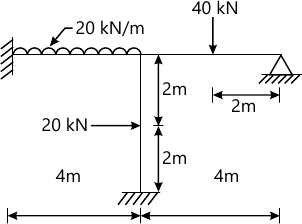20 M

3 Analyze the continuous beam ABCD 20m long simply supported at its ends and loaded as shown in Fig. Q3. If support B sinks by 10 mm, analyze the beam by moment distribution method. Sketch the SFD and BMD. Take E=2.1×105 N/mm2 and I=85×105 mm4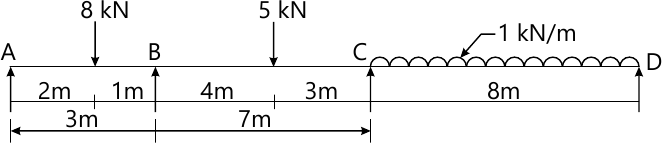20 M

4 Analyze the frame shown in fig Q4 by moment distribution method.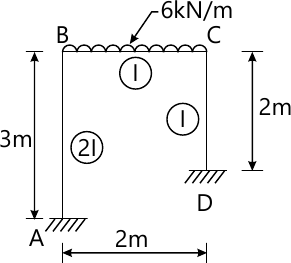20 M

5 Analyze the beam loaded as shown in Fig Q5 using Kani's method. Draw BMD.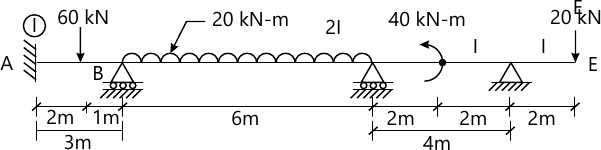20 M

6 Analyze the portal frame ABCD shown in Fig Q6 by flexibility method. EI is constant throughout.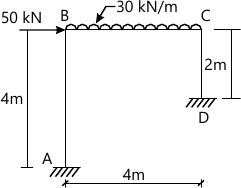20 M

7 Analyze the frame shown in Fig Q7 by stiffness matrix method. Draw BMD and elastic curve.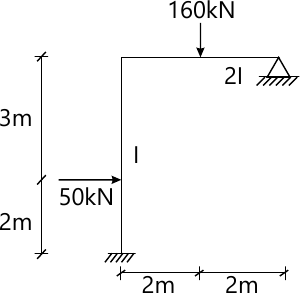20 M

8 (a) Explain the following:
i) Vibration and oscillation
ii) Free vibration and forced vibration.
iii) Damping and types of damping.
iv) Degree of freedom and single degree of freedom system.
12 M
8 (b) Calculate the natural angular frequency in sideway for the frame in Fig. Q8 (b) and also the natural period of vibration. If the initial displacement is 25 mm and the initial velocity is 25 mm/s, what is the amplitude and displacement at t=1 sec.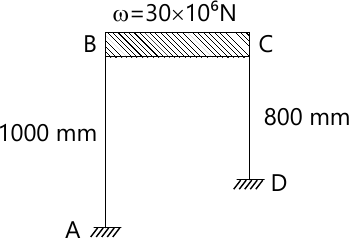EI(AB) = EI(CD) = 30 × 1012 N-mm2

8 M

More question papers from Structural Analysis 2